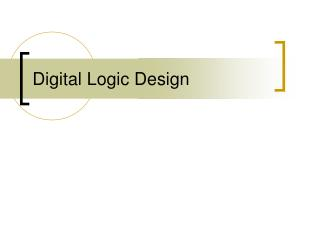DownloadDownload PresentationDigital Logic Design

# Digital Logic Design

Download Presentation## Digital Logic Design

- - - - - - - - - - - - - - - - - - - - - - - - - - - E N D - - - - - - - - - - - - - - - - - - - - - - - - - - -
##### Presentation Transcript

1. Digital Logic Design

2. Truth Table  Logic Circuit • Start with truth table • When your output is a 1, figure out the combination of inputs, ANDs, and NOTs Example: A = 0, B = 1, C = 0  A’·B·C’ A = 1, B = 1, C = 0  A·B·C’ • When you have all your “miniterms,” OR all of them together Example: f = A’·B·C’ + A·B·C’ • Simplify your boolean expression • Build your digital circuit

3. Example • What is the boolean function for the truth table below? miniterms: A’·B’·C A’·B·C A·B’·C’ A·B’·C A·B·C f = A’·B’·C + A’·B·C + A·B’·C’ + A·B’·C + A·B·C

4. Example • Simplify the boolean expression f = A’·B’·C + A’·B·C + A·B’·C’ + A·B’·C + A·B·C f = A’·C + A·B’ + A·B·C f = A’·C + A ( B’ + C ) • Build the digital circuit from the simplified expression

5. Your Turn • Design the digital circuit for the following truth table:

6. 1) Get the Boolean expression? Hint: What are the miniterms?

7. 2) Simplify! f = A’·B·C + A·B’·C’ + A·B’·C + A·B·C’ + A·B·C

8. 3) Build the Digital Circuit f = A + B·C

9. Done! f = A + B·C